# NCERT Solutions for Class 10 Science Chapter 12 – Electricity

NCERT Solutions for Class 10 Science Chapter 12 – Electricity, contains solutions to various questions in Exercises for Chapter 12. Electricity Class 10 NCERT Solutions have been explained in a simple and easy to understand manner. We are providing all subjects which can be accessed by clicking here.

## Download NCERT Solutions for Class 10 Science Chapter 12 – Electricity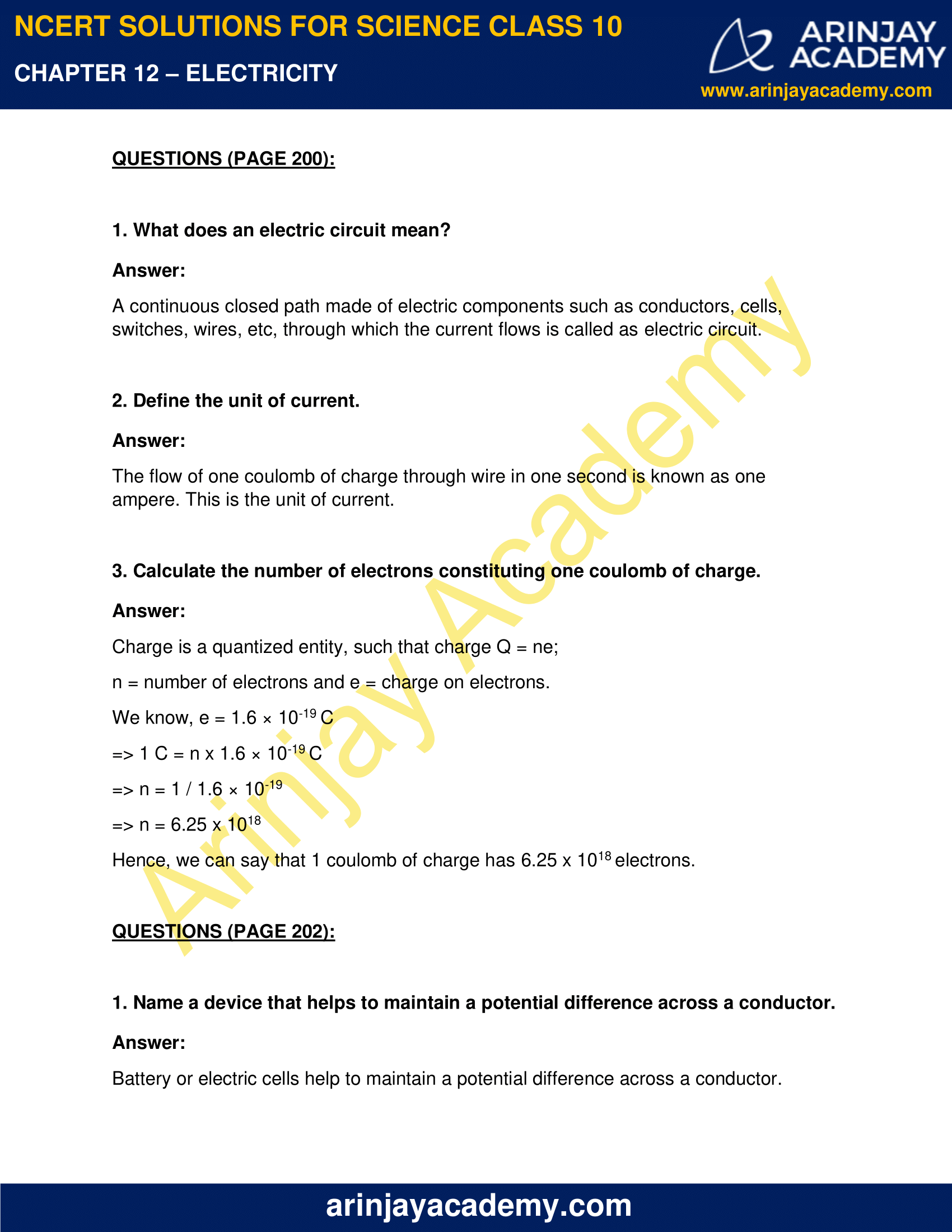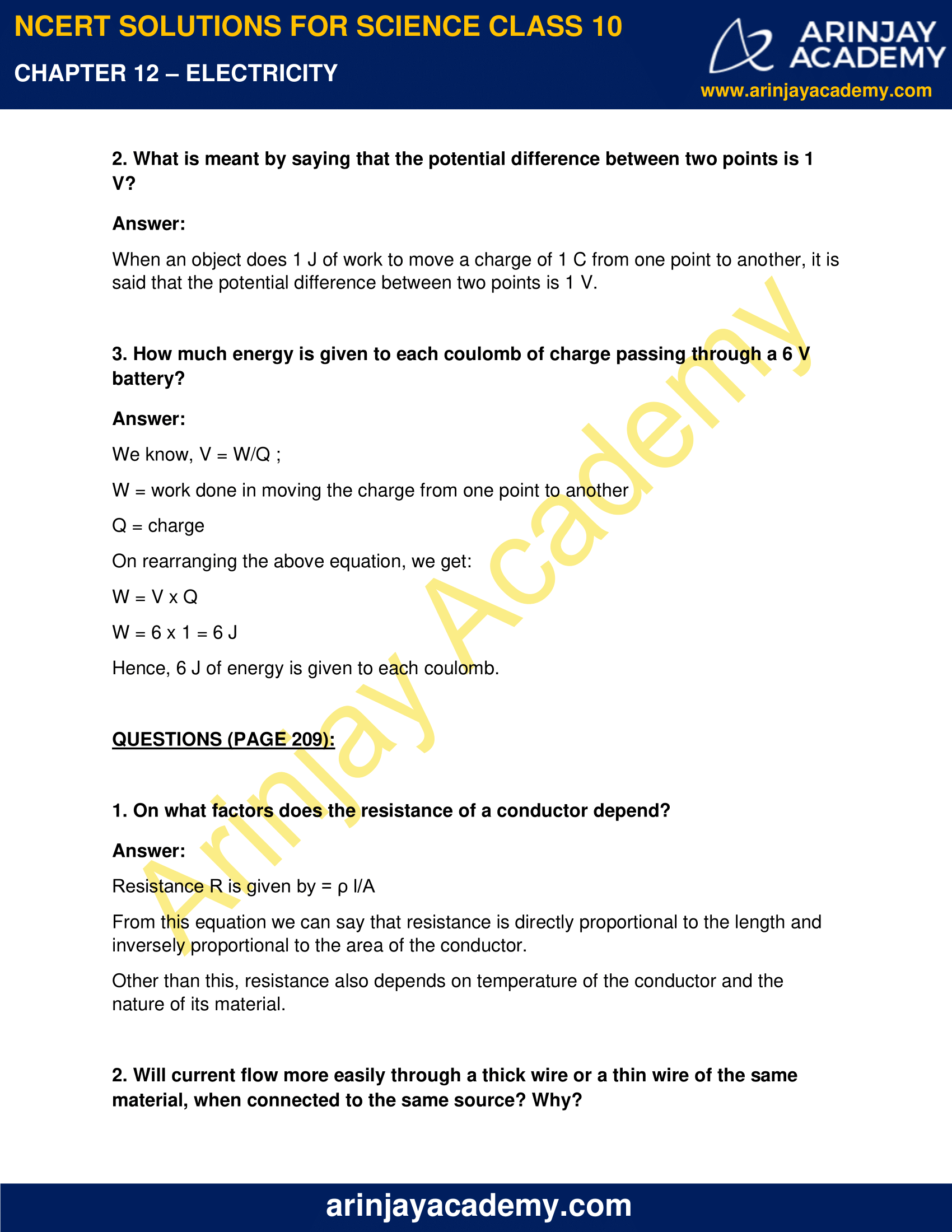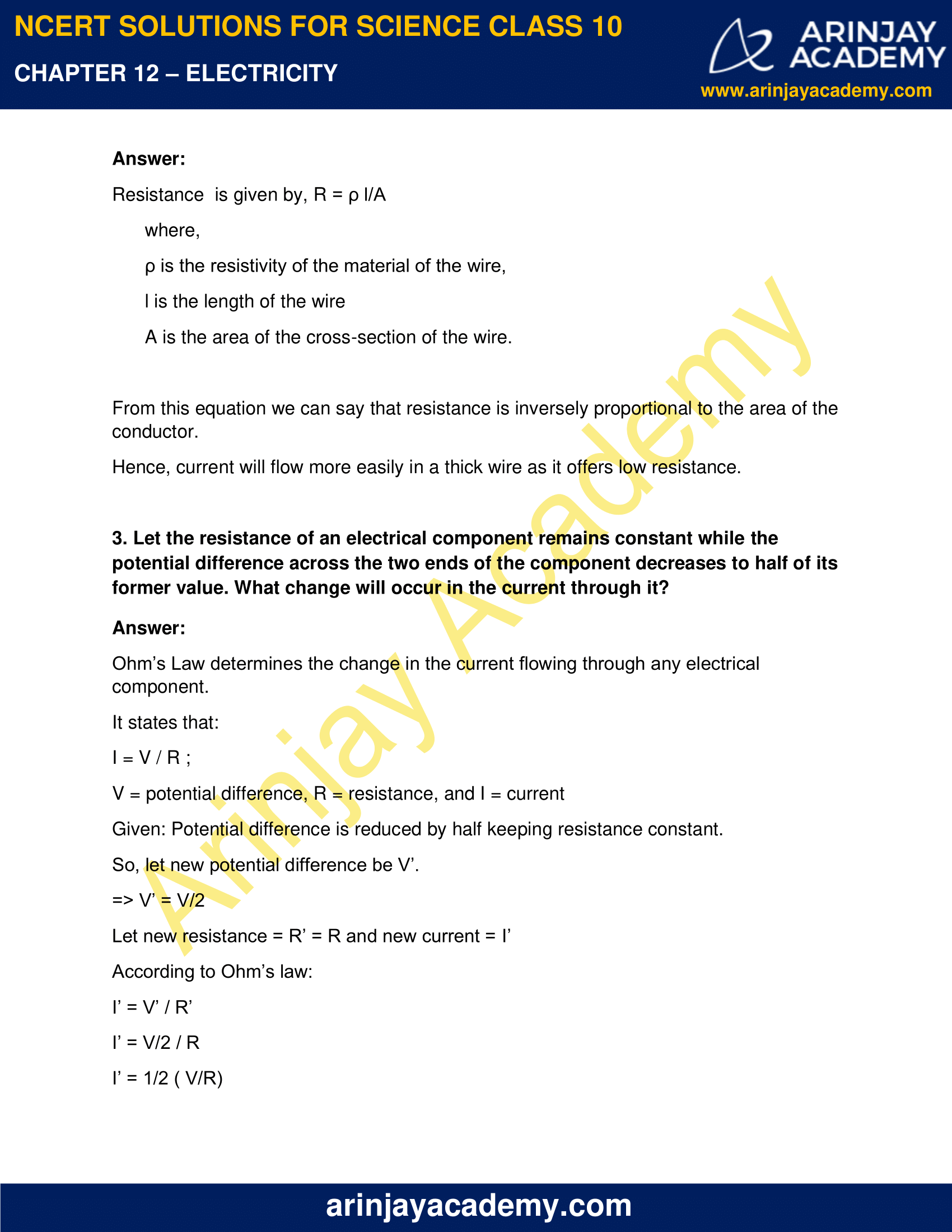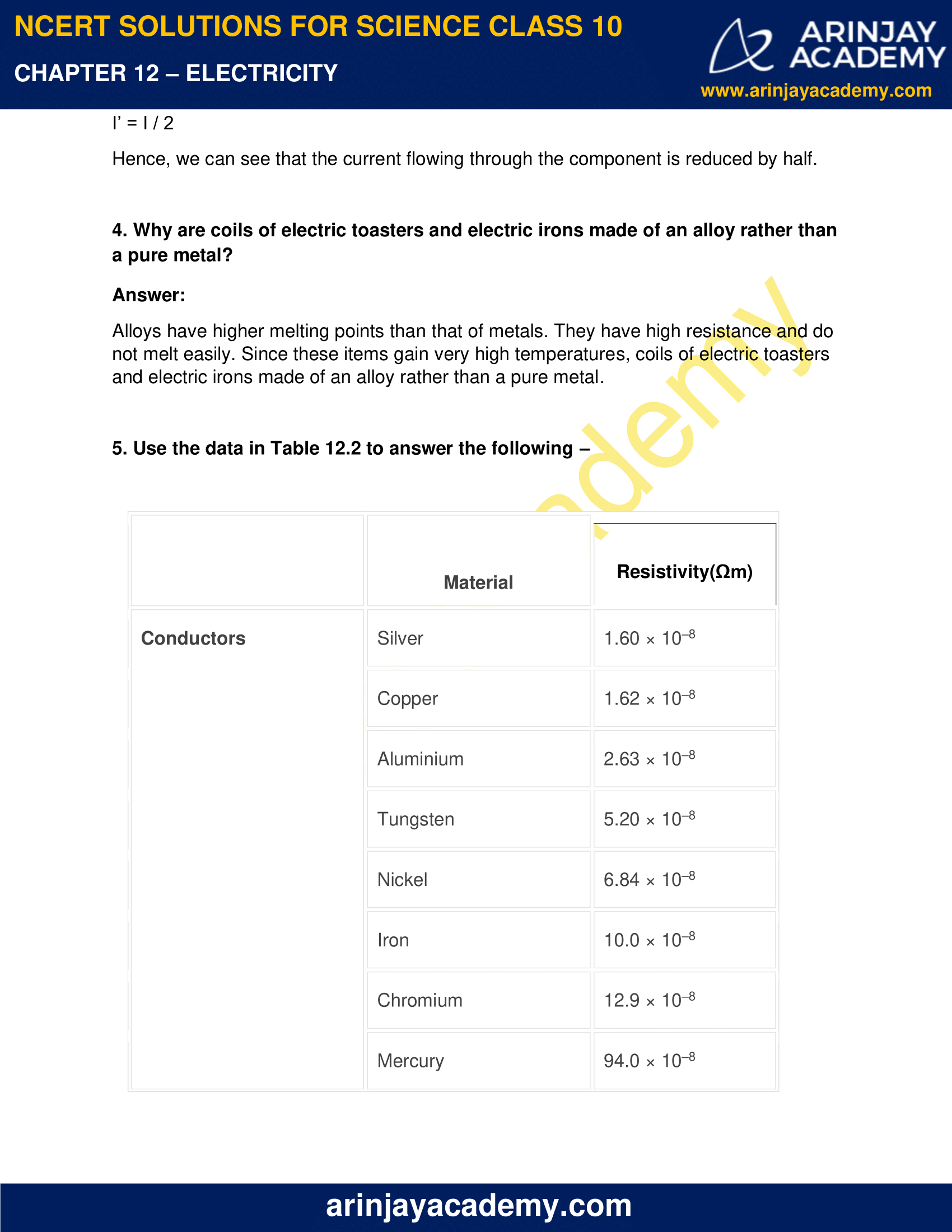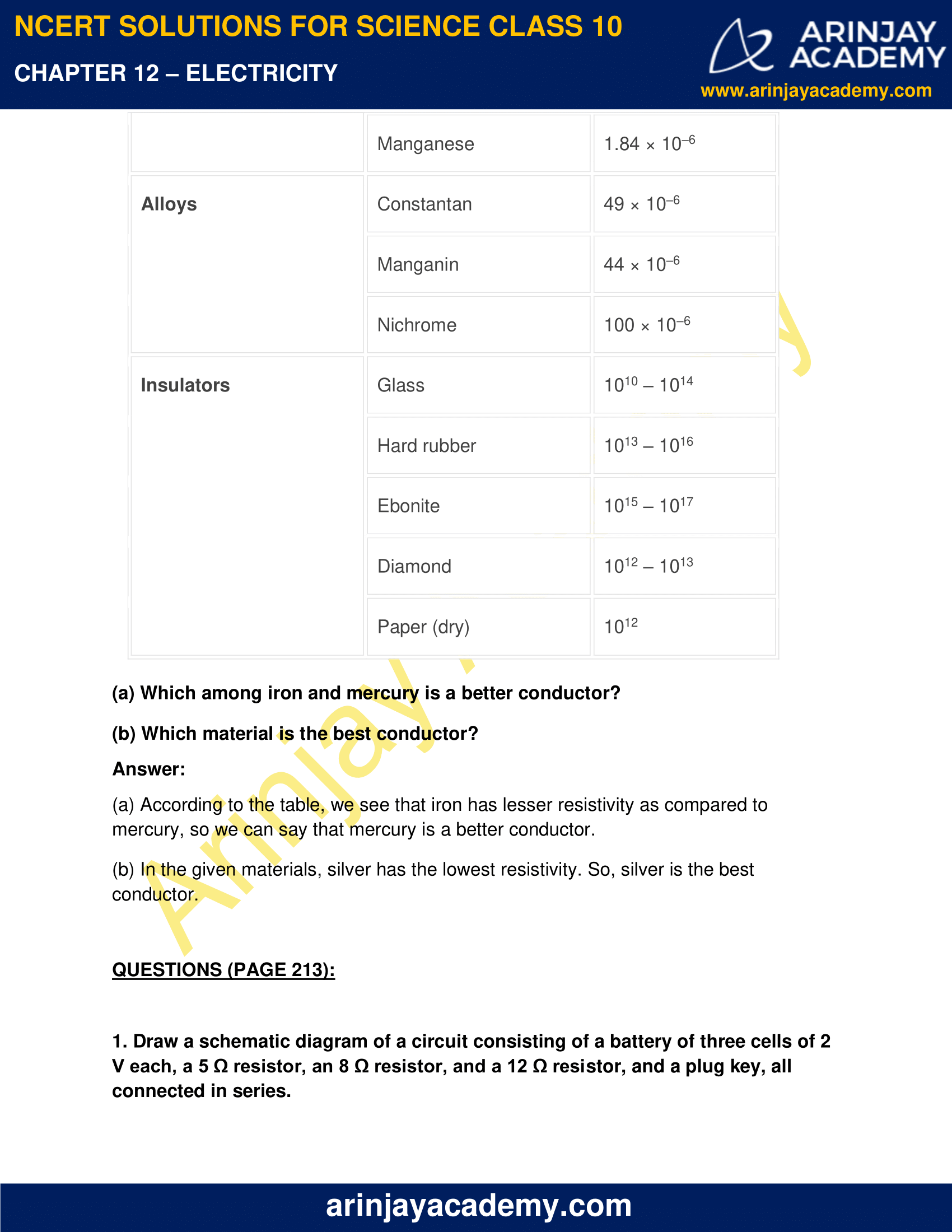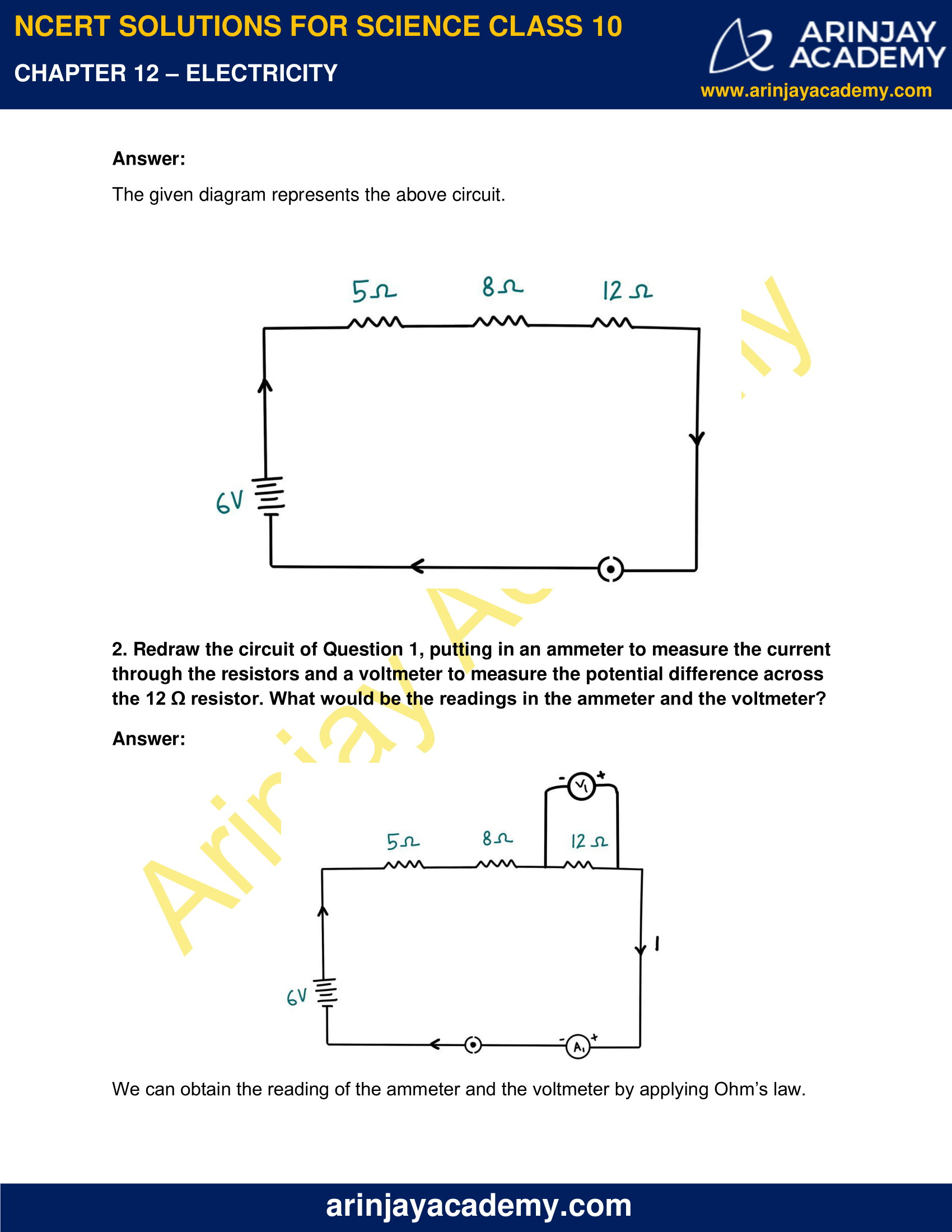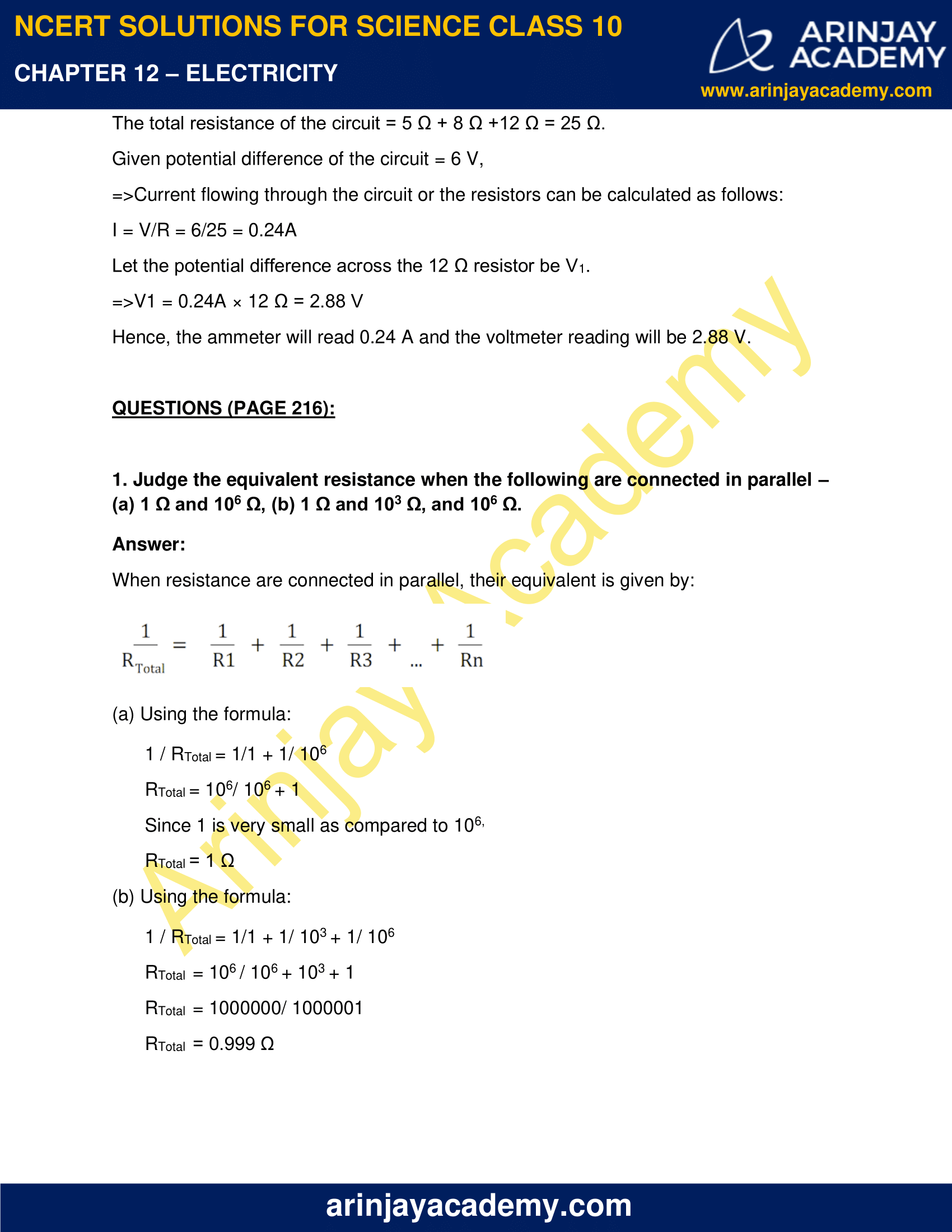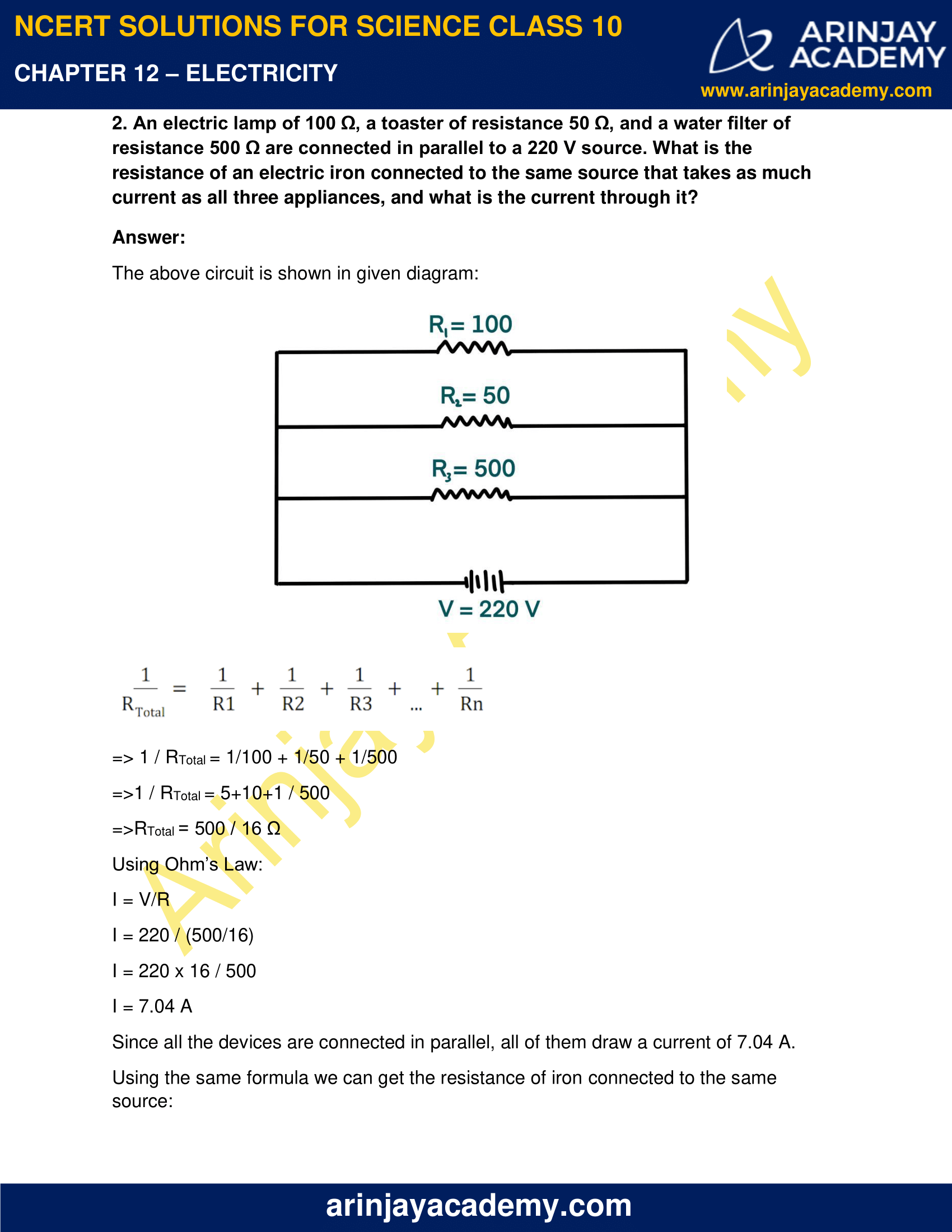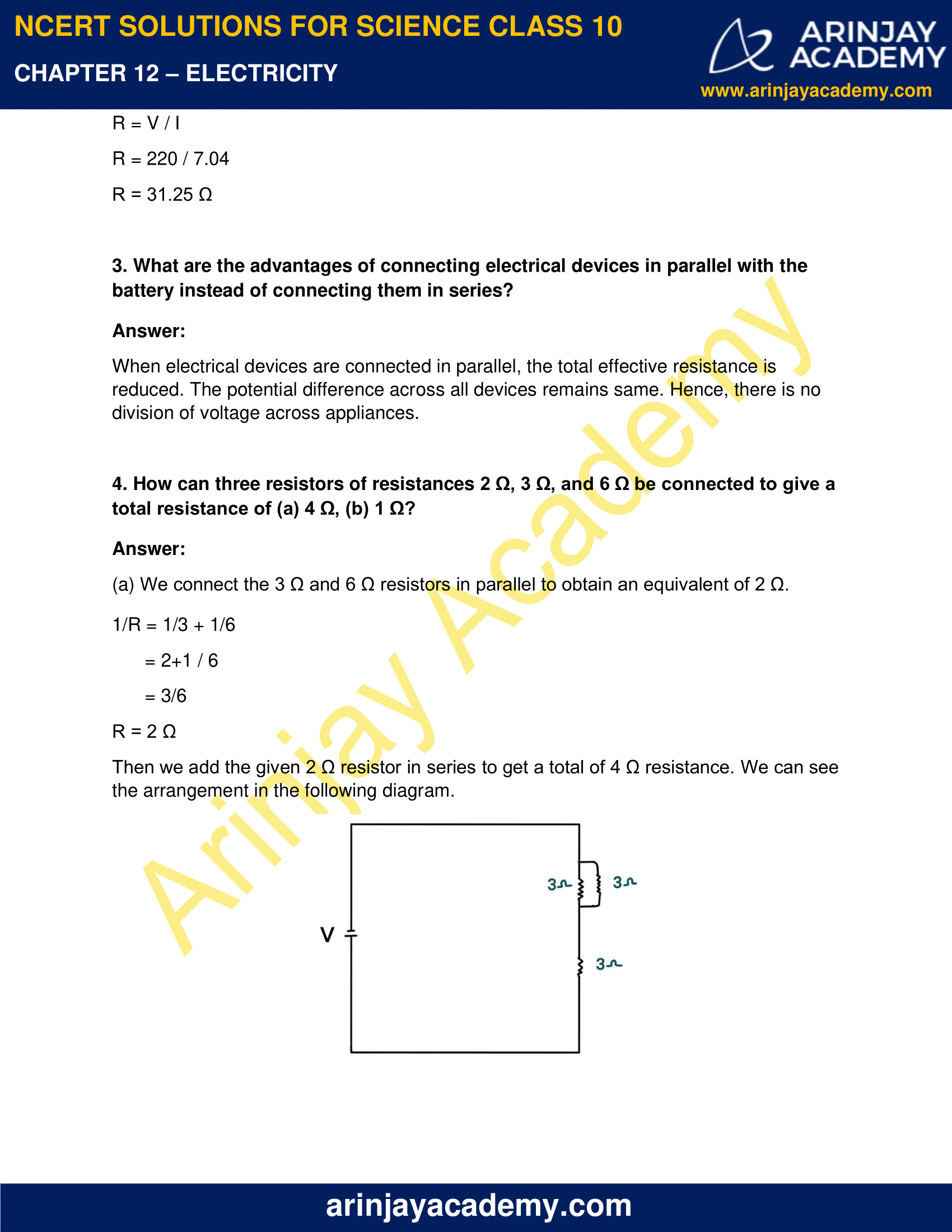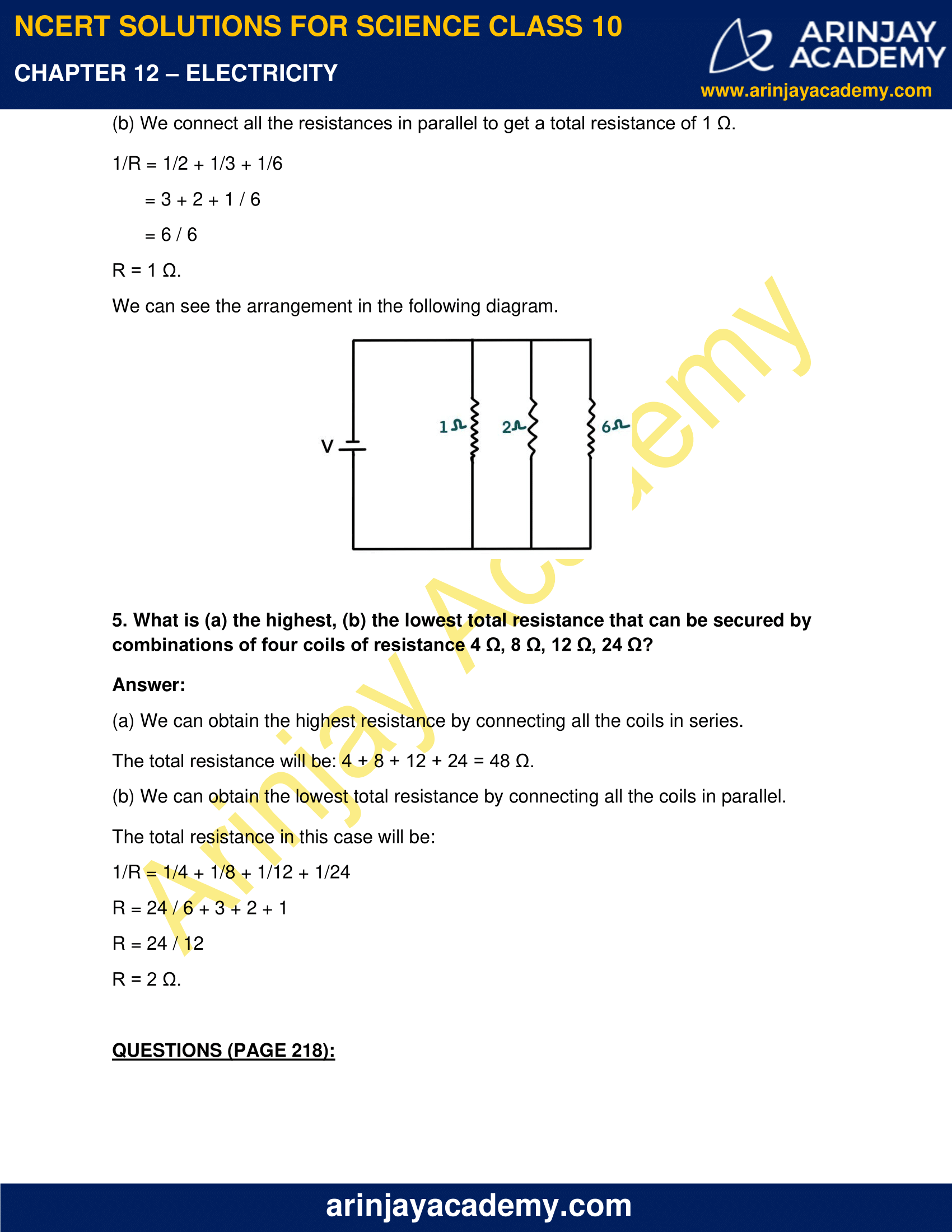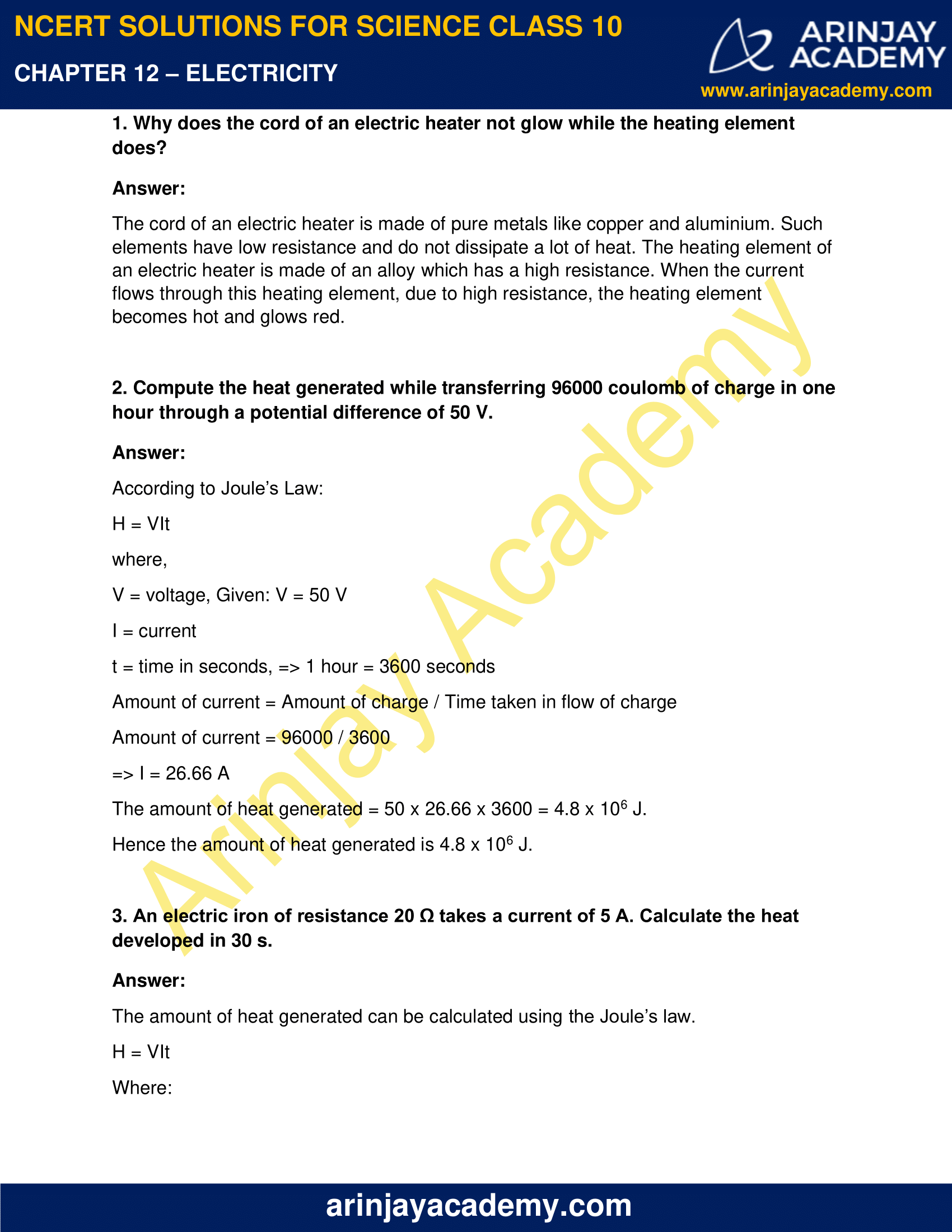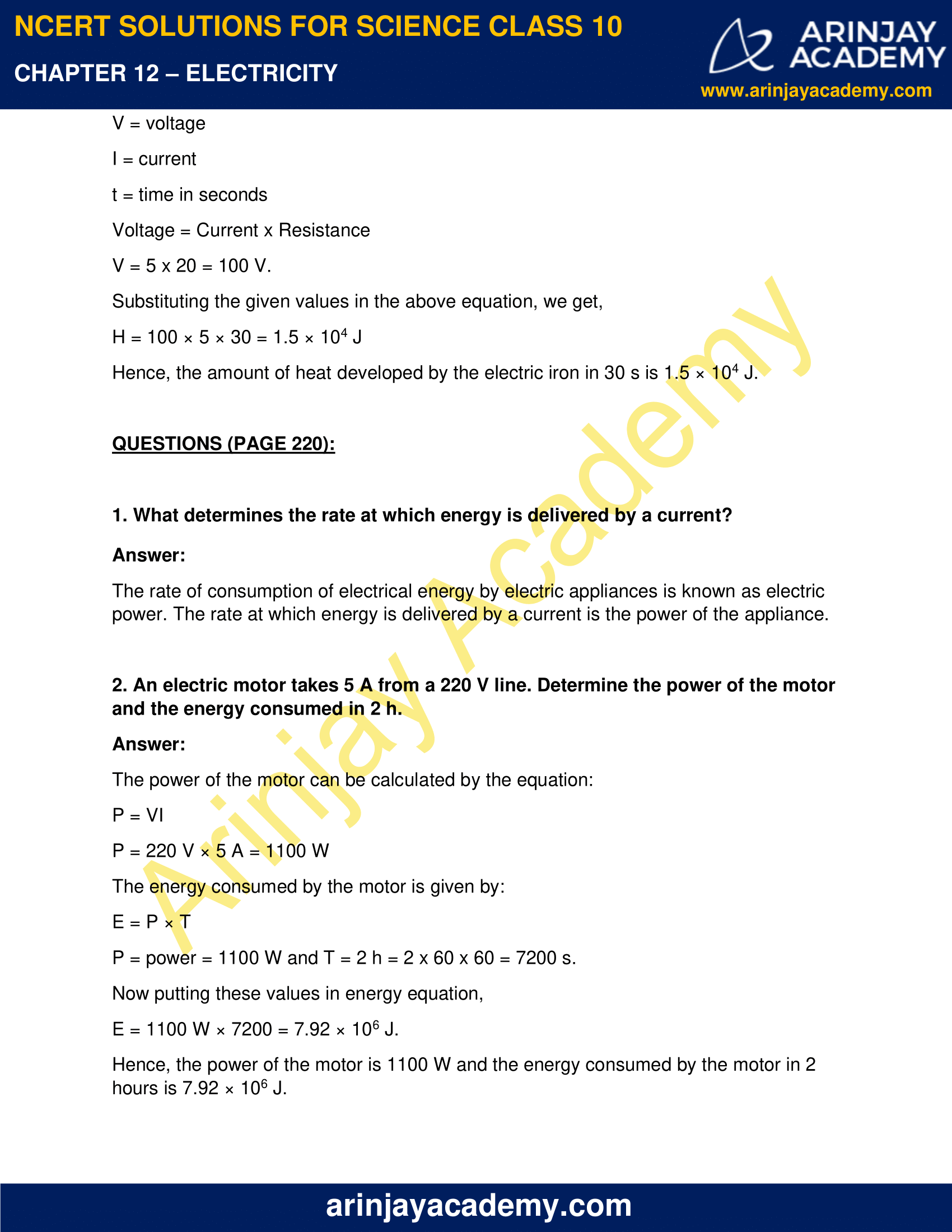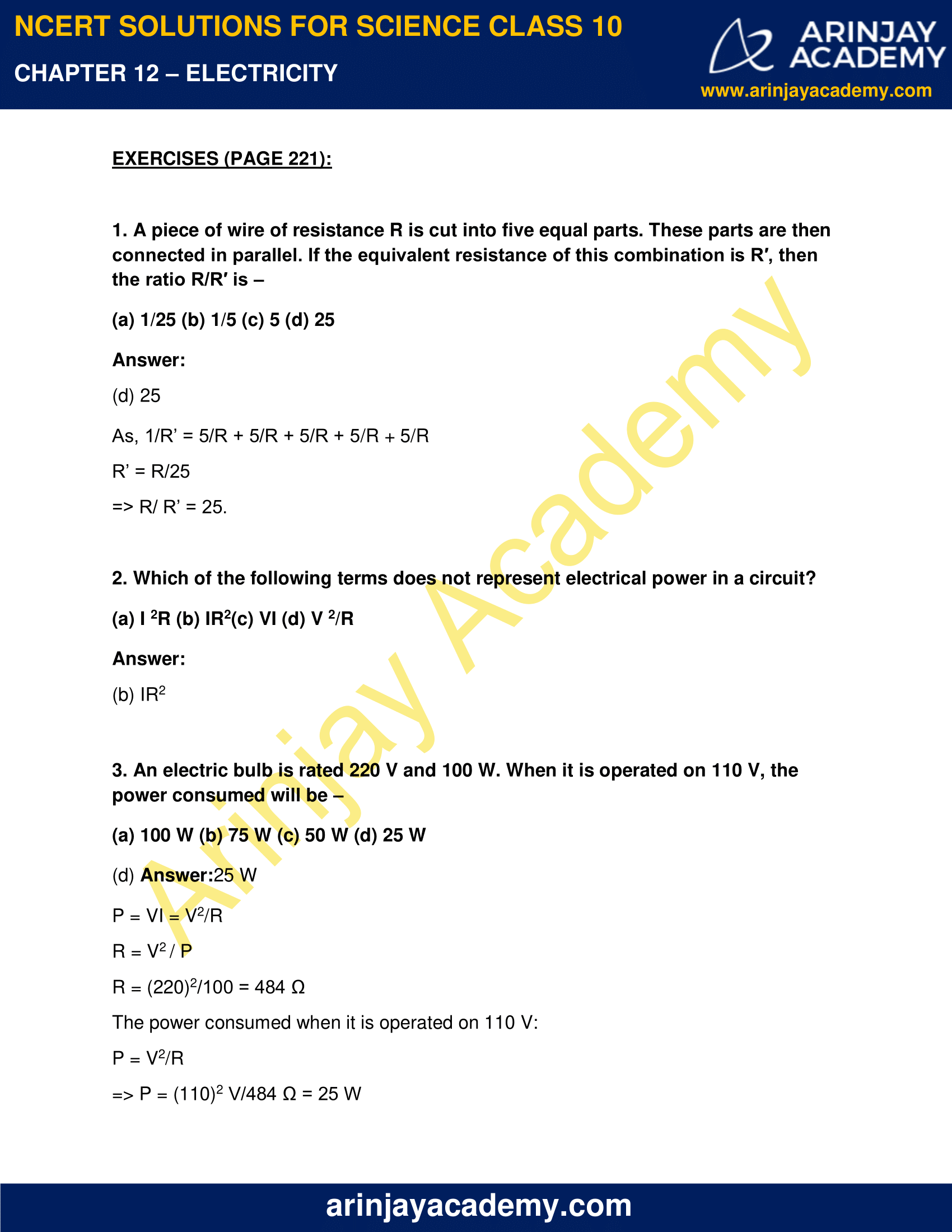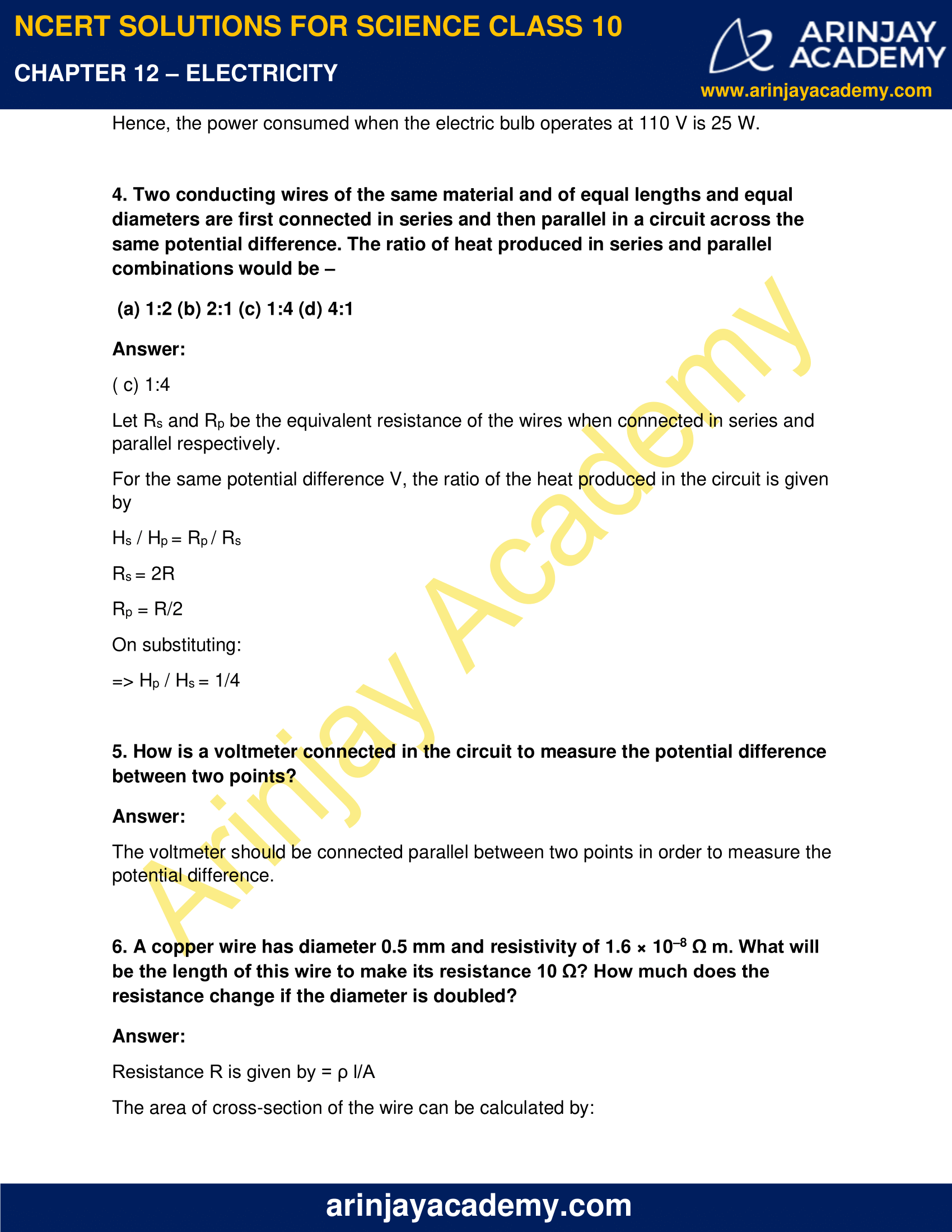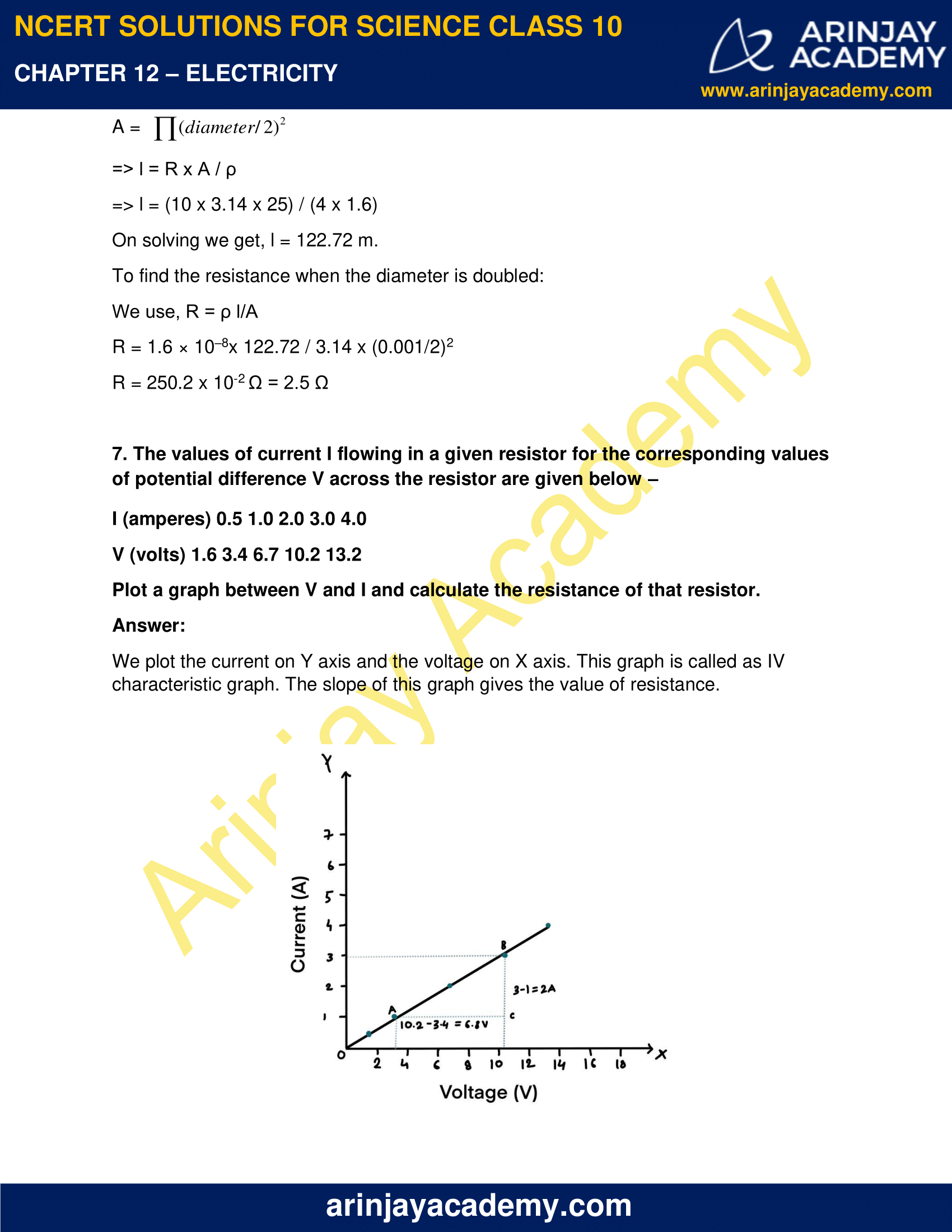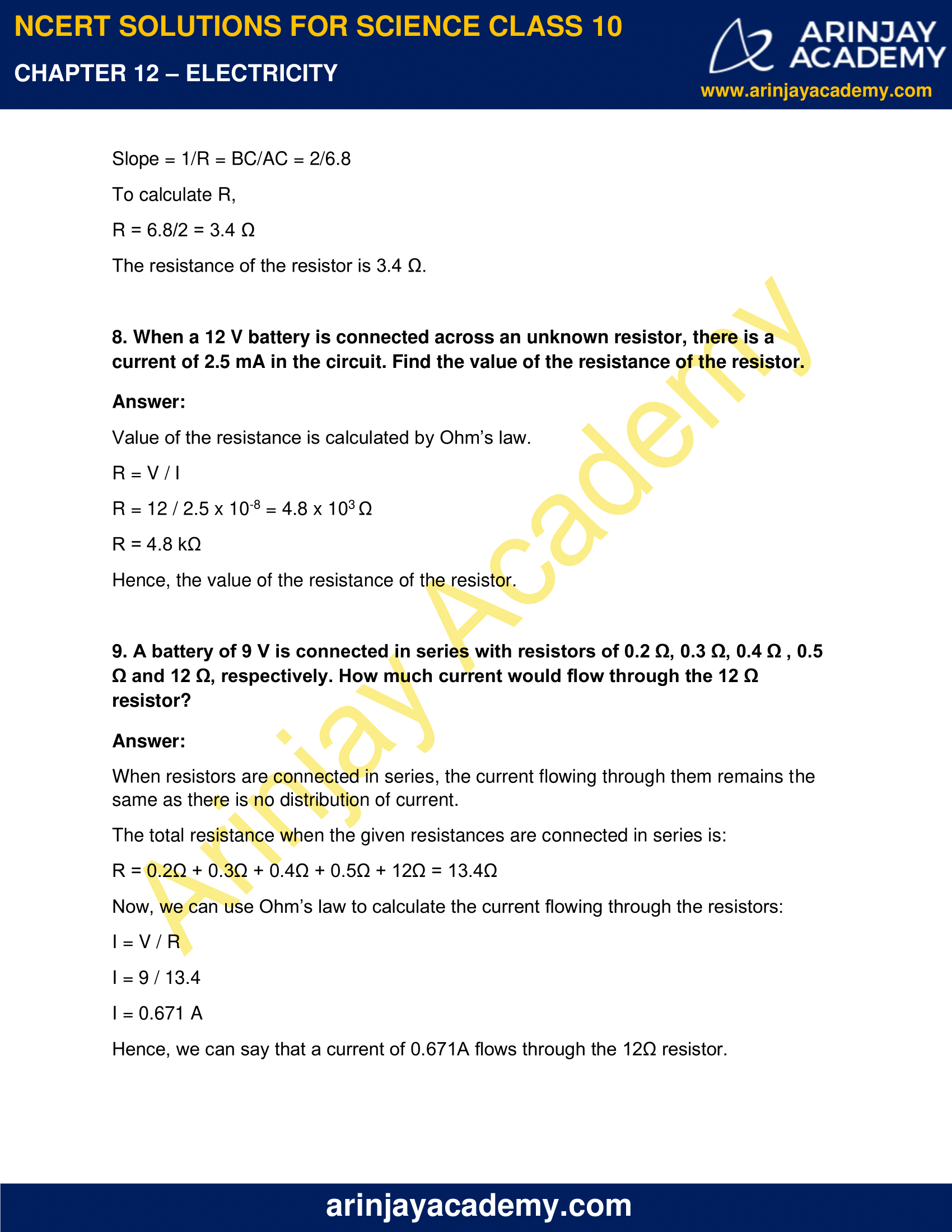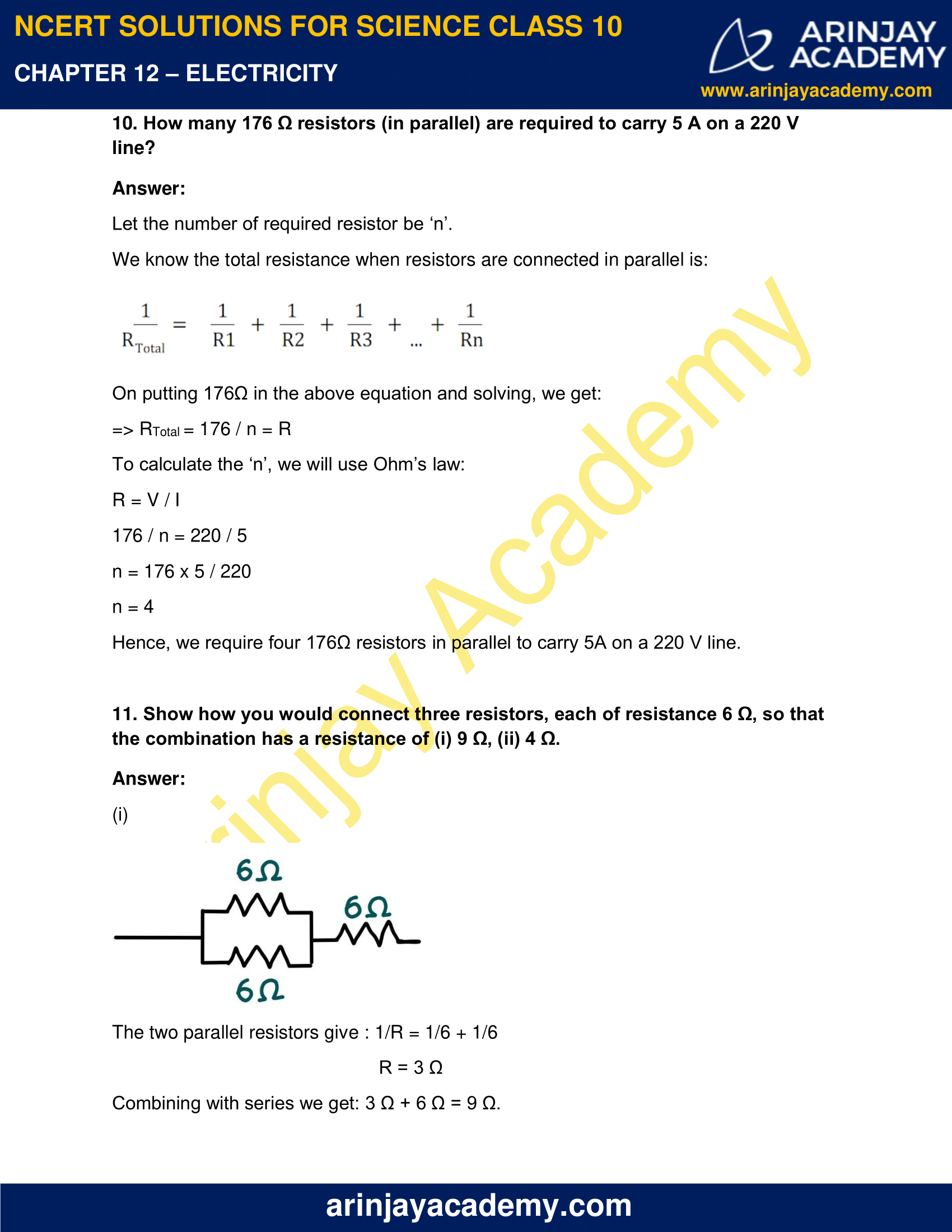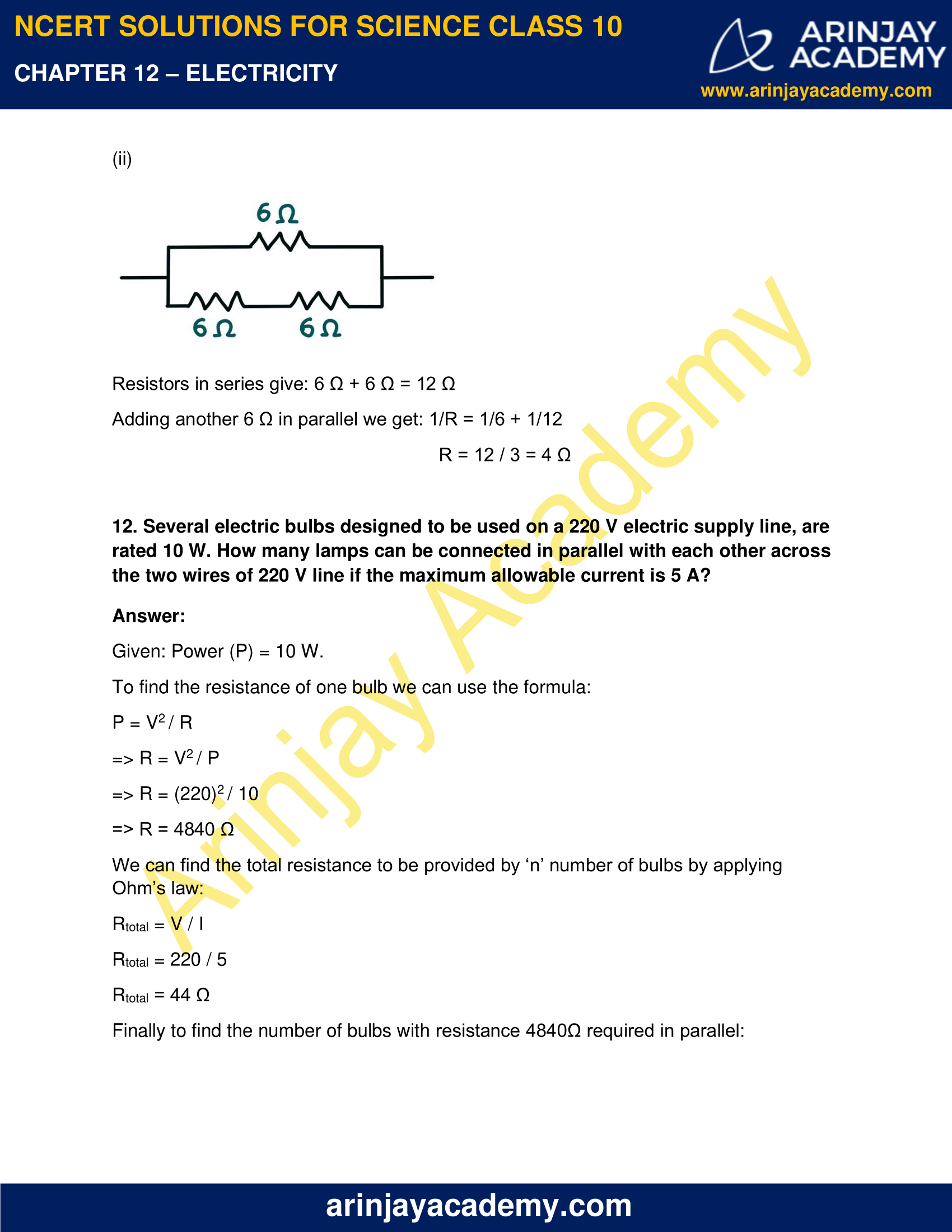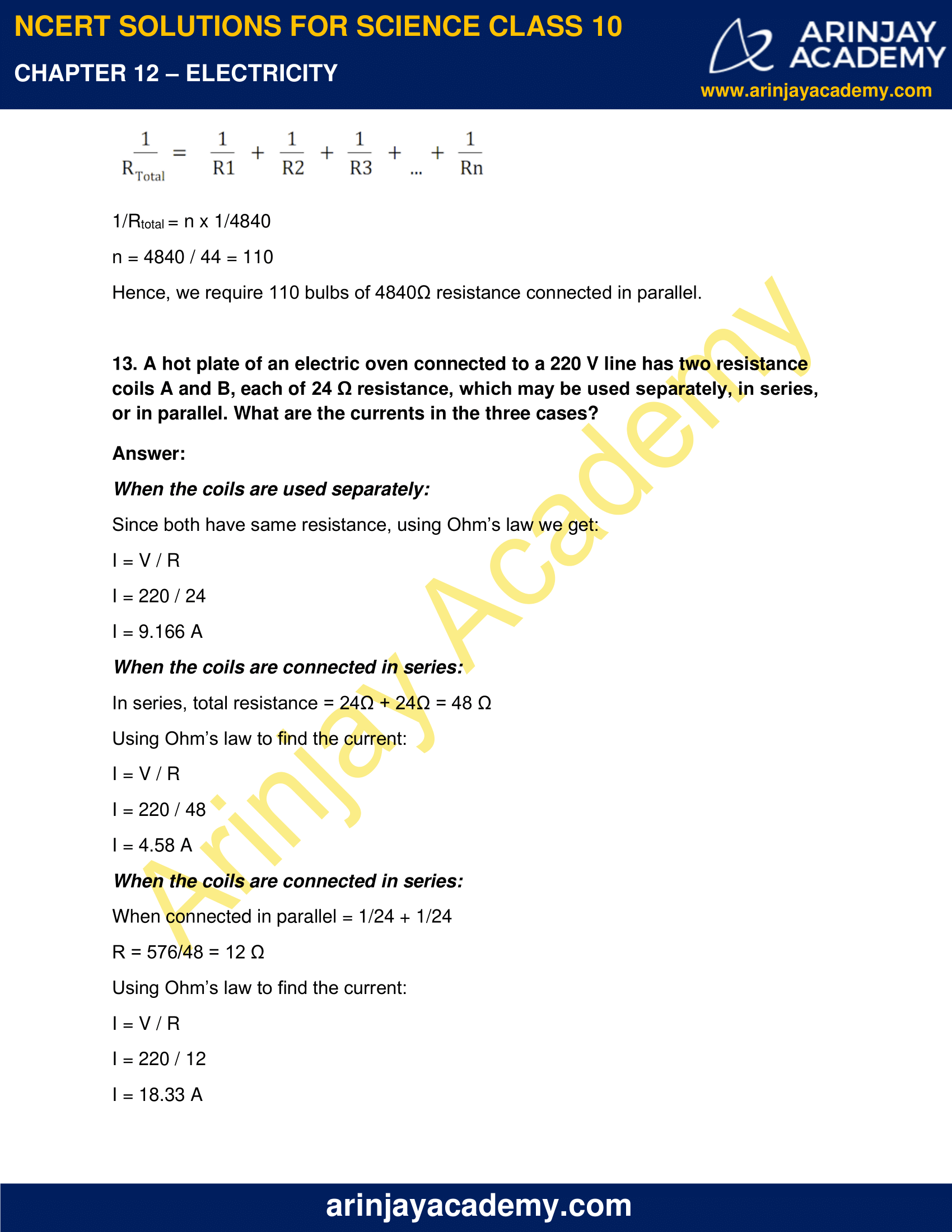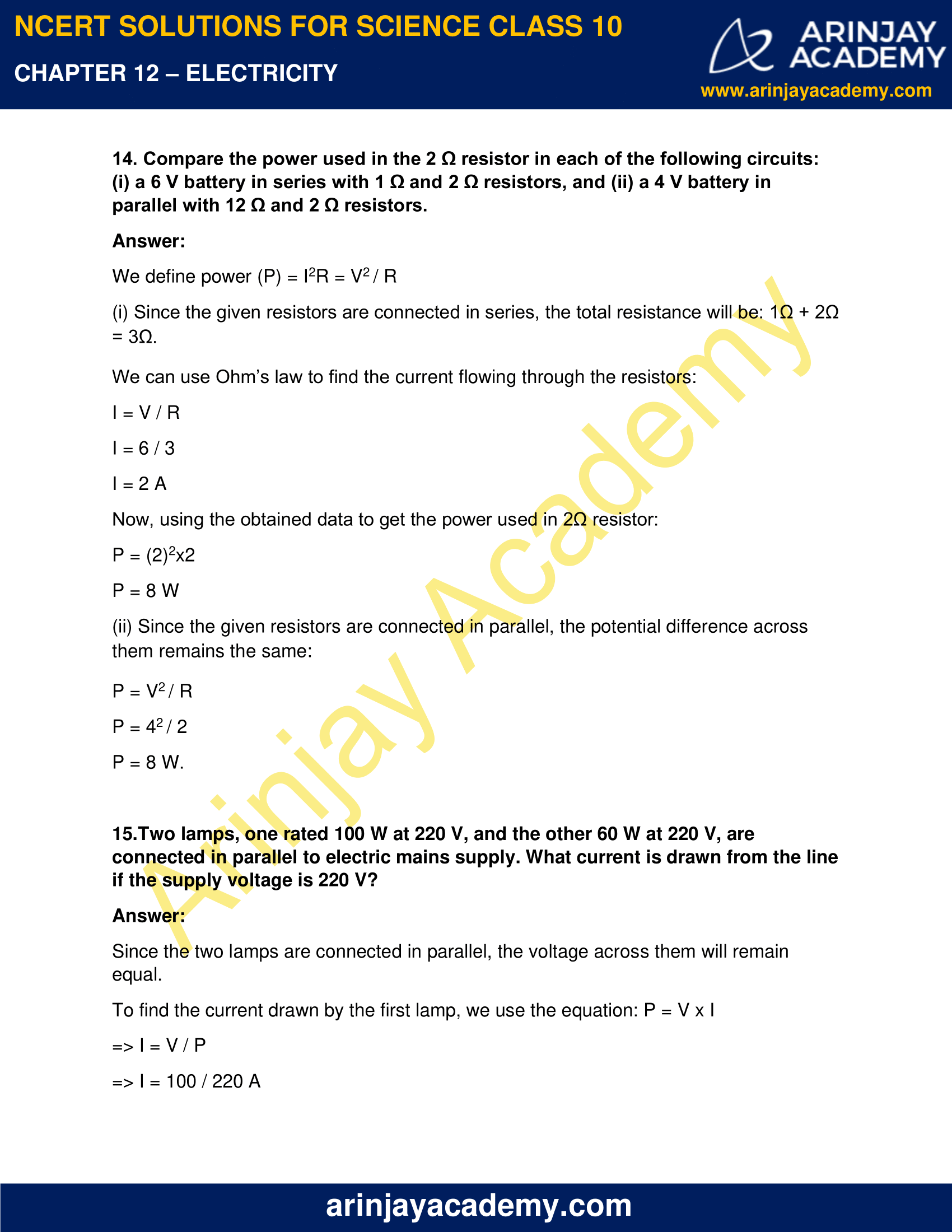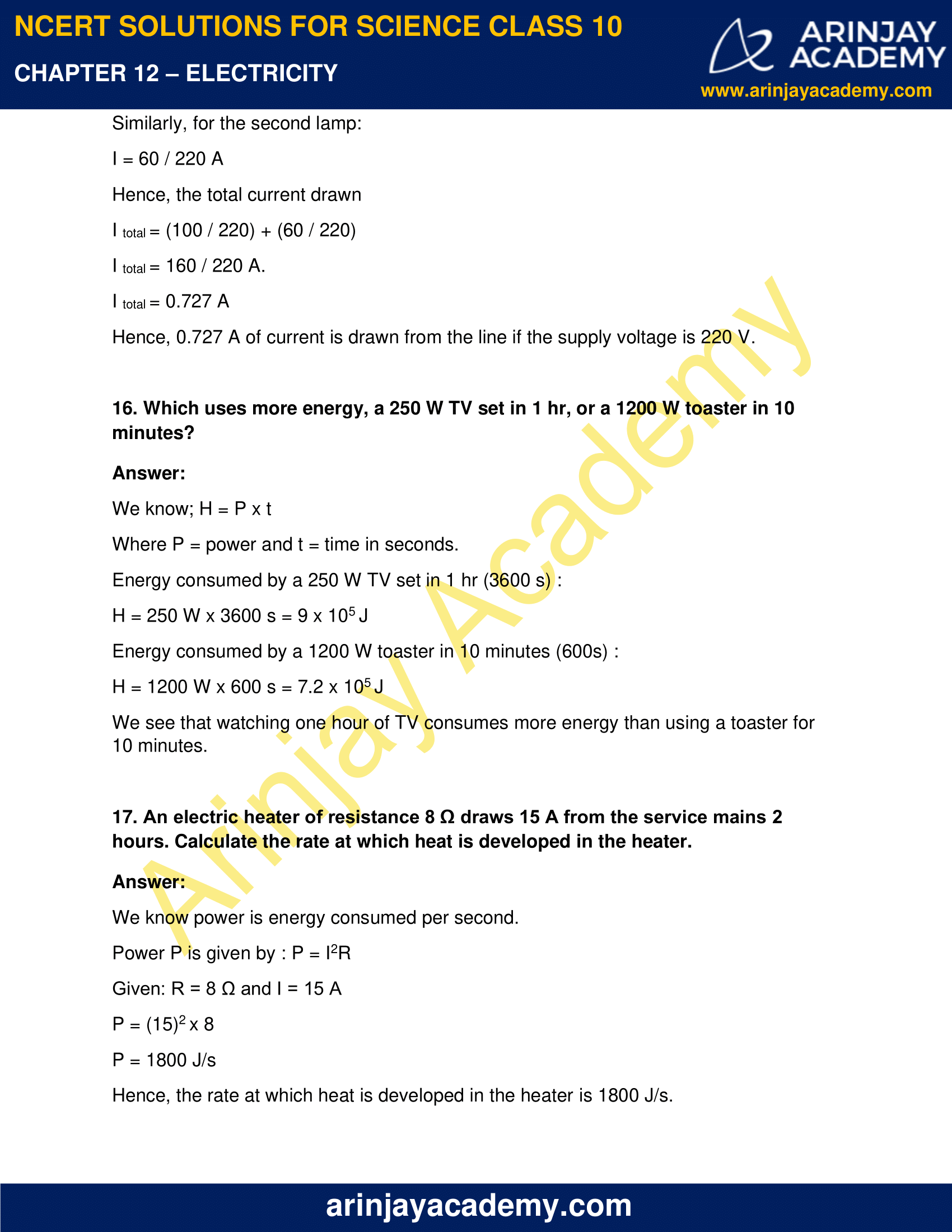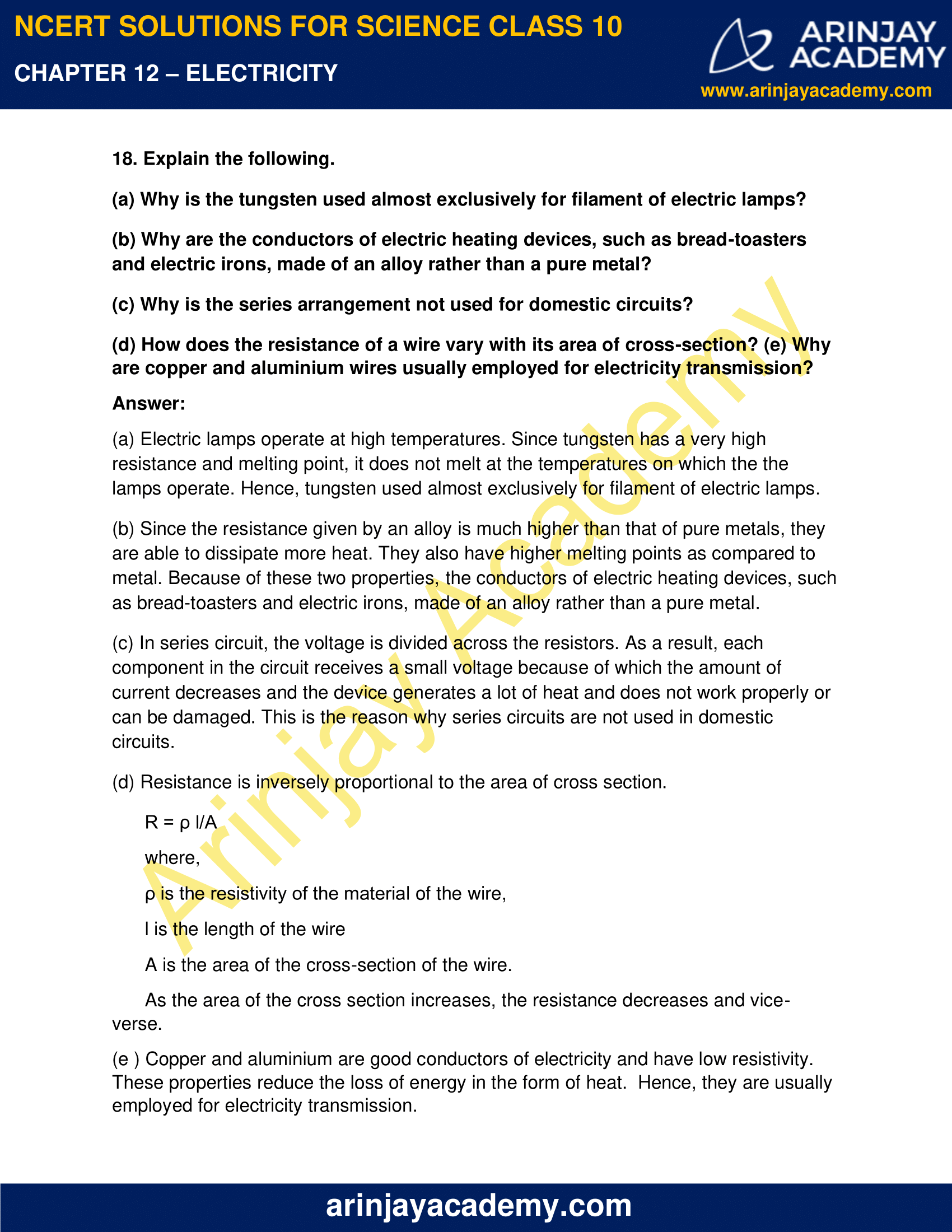### NCERT Solutions for Class 10 Science Chapter 12 – Electricity – NCERT Exercises

NCERT Solutions for Class 10 Science Chapter 12 – Electricity, Exercises includes all in text and exercise solved questions which helps you to understand the topic covered in Chapter 12 Electricity Class 10, in a better manner to help you to score good marks in your examinations.

### NCERT Solutions for Class 10 Science Chapter 12 – Intext Exercise – Page 200

1. What does an electric circuit mean?

Answer:

A continuous closed path made of electric components such as conductors, cells, switches, wires, etc, through which the current flows is called as electric circuit.

2. Define the unit of current.

Answer:

The flow of one coulomb of charge through wire in one second is known as one ampere. This is the unit of current.

3. Calculate the number of electrons constituting one coulomb of charge.

Answer:

Charge is a quantized entity, such that charge Q = ne;

n = number of electrons and e = charge on electrons.

We know, e = 1.6 × 10-19 C

=> 1 C = n x 1.6 × 10-19 C

=> n = 1 / 1.6 × 10-19

=> n = 6.25 x 1018

Hence, we can say that 1 coulomb of charge has 6.25 x 1018 electrons.

### Intext Exercise – Page 202

1. Name a device that helps to maintain a potential difference across a conductor.

Answer:

Battery or electric cells help to maintain a potential difference across a conductor.

2. What is meant by saying that the potential difference between two points is 1 V?

Answer:

When an object does 1 J of work to move a charge of 1 C from one point to another, it is said that the potential difference between two points is 1 V.

3. How much energy is given to each coulomb of charge passing through a 6 V battery?

Answer:

We know, V = W/Q ;

W = work done in moving the charge from one point to another

Q = charge

On rearranging the above equation, we get:

W = V x Q

W = 6 x 1 = 6 J

Hence, 6 J of energy is given to each coulomb.

### Intext Exercise – Page 209

1. On what factors does the resistance of a conductor depend?

Answer:

Resistance R is given by = ρ l/A

From this equation we can say that resistance is directly proportional to the length and inversely proportional to the area of the conductor.

Other than this, resistance also depends on temperature of the conductor and the nature of its material.

2. Will current flow more easily through a thick wire or a thin wire of the same material, when connected to the same source? Why?

Answer:

Resistance  is given by, R = ρ l/A

where,

ρ is the resistivity of the material of the wire,

l is the length of the wire

A is the area of the cross-section of the wire.

From this equation we can say that resistance is inversely proportional to the area of the conductor.

Hence, current will flow more easily in a thick wire as it offers low resistance.

3. Let the resistance of an electrical component remains constant while the potential difference across the two ends of the component decreases to half of its former value. What change will occur in the current through it?

Answer:

Ohm’s Law determines the change in the current flowing through any electrical component.

It states that:

I = V / R ;

V = potential difference, R = resistance, and I = current

Given: Potential difference is reduced by half keeping resistance constant.

So, let new potential difference be V’.

=> V’ = V/2

Let new resistance = R’ = R and new current = I’

According to Ohm’s law:

I’ = V’ / R’

I’ = V/2 / R

I’ = 1/2 ( V/R)

I’ = I / 2

Hence, we can see that the current flowing through the component is reduced by half.

4. Why are coils of electric toasters and electric irons made of an alloy rather than a pure metal?

Answer:

Alloys have higher melting points than that of metals. They have high resistance and do not melt easily. Since these items gain very high temperatures, coils of electric toasters and electric irons made of an alloy rather than a pure metal.

5. Use the data in Table 12.2 to answer the following –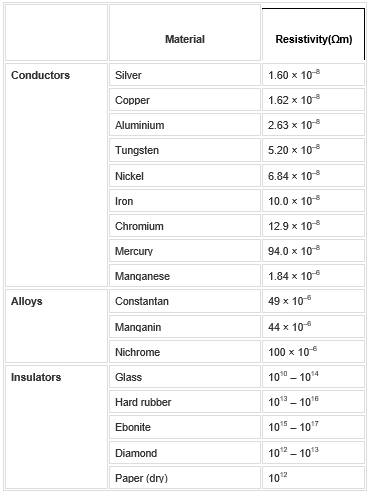(a) Which among iron and mercury is a better conductor?

(b) Which material is the best conductor?

Answer:

(a) According to the table, we see that iron has lesser resistivity as compared to mercury, so we can say that mercury is a better conductor.

(b) In the given materials, silver has the lowest resistivity. So, silver is the best conductor.

QUESTIONS (PAGE 213):

1. Draw a schematic diagram of a circuit consisting of a battery of three cells of 2 V each, a 5 Ω resistor, an 8 Ω resistor, and a 12 Ω resistor, and a plug key, all connected in series.

Answer:

The given diagram represents the above circuit.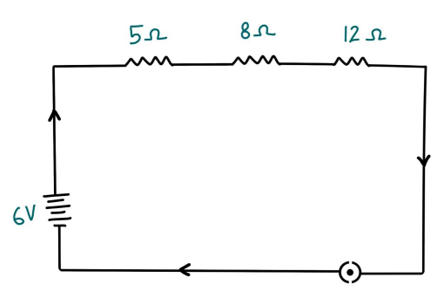2. Redraw the circuit of Question 1, putting in an ammeter to measure the current through the resistors and a voltmeter to measure the potential difference across the 12 Ω resistor. What would be the readings in the ammeter and the voltmeter?

Answer: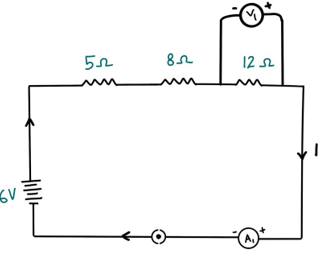We can obtain the reading of the ammeter and the voltmeter by applying Ohm’s law.

The total resistance of the circuit = 5 Ω + 8 Ω +12 Ω = 25 Ω.

Given potential difference of the circuit = 6 V,

=>Current flowing through the circuit or the resistors can be calculated as follows:

I = V/R = 6/25 = 0.24A

Let the potential difference across the 12 Ω resistor be V1.

=>V1 = 0.24A × 12 Ω = 2.88 V

Hence, the ammeter will read 0.24 A and the voltmeter reading will be 2.88 V.

### NCERT Solutions for Class 10 Science Chapter 12 – Intext Exercise – Page 216

1. Judge the equivalent resistance when the following are connected in parallel – (a) 1 Ω and 106 Ω, (b) 1 Ω and 103 Ω, and 106 Ω.

Answer:

When resistance are connected in parallel, their equivalent is given by: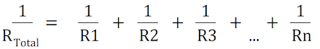(a) Using the formula:

1 / RTotal = 1/1 + 1/ 106

RTotal = 106/ 106 + 1

Since 1 is very small as compared to 106,

RTotal = 1 Ω

(b) Using the formula:

1 / RTotal = 1/1 + 1/ 103 + 1/ 106

RTotal  = 106 / 106 + 103 + 1

RTotal  = 1000000/ 1000001

RTotal  = 0.999 Ω

2. An electric lamp of 100 Ω, a toaster of resistance 50 Ω, and a water filter of resistance 500 Ω are connected in parallel to a 220 V source. What is the resistance of an electric iron connected to the same source that takes as much current as all three appliances, and what is the current through it?

Answer:

The above circuit is shown in given diagram: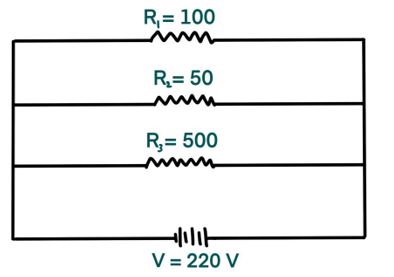=> 1 / RTotal = 1/100 + 1/50 + 1/500

=>1 / RTotal = 5+10+1 / 500

=>RTotal = 500 / 16 Ω

Using Ohm’s Law:

I = V/R

I = 220 / (500/16)

I = 220 x 16 / 500

I = 7.04 A

Since all the devices are connected in parallel, all of them draw a current of 7.04 A.

Using the same formula we can get the resistance of iron connected to the same source:

R = V / I

R = 220 / 7.04

R = 31.25 Ω

3. What are the advantages of connecting electrical devices in parallel with the battery instead of connecting them in series?

Answer:

When electrical devices are connected in parallel, the total effective resistance is reduced. The potential difference across all devices remains same. Hence, there is no division of voltage across appliances.

4. How can three resistors of resistances 2 Ω, 3 Ω, and 6 Ω be connected to give a total resistance of (a) 4 Ω, (b) 1 Ω?

Answer:

(a) We connect the 3 Ω and 6 Ω resistors in parallel to obtain an equivalent of 2 Ω.

1/R = 1/3 + 1/6

= 2+1 / 6

= 3/6

R = 2 Ω

Then we add the given 2 Ω resistor in series to get a total of 4 Ω resistance. We can see the arrangement in the following diagram.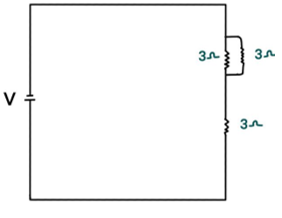(b) We connect all the resistances in parallel to get a total resistance of 1 Ω.

1/R = 1/2 + 1/3 + 1/6

= 3 + 2 + 1 / 6

= 6 / 6

R = 1 Ω.

We can see the arrangement in the following diagram.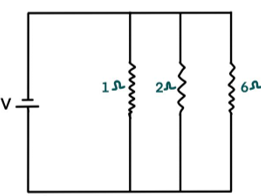5. What is (a) the highest, (b) the lowest total resistance that can be secured by combinations of four coils of resistance 4 Ω, 8 Ω, 12 Ω, 24 Ω?

Answer:

(a) We can obtain the highest resistance by connecting all the coils in series.

The total resistance will be: 4 + 8 + 12 + 24 = 48 Ω.

(b) We can obtain the lowest total resistance by connecting all the coils in parallel.

The total resistance in this case will be:

1/R = 1/4 + 1/8 + 1/12 + 1/24

R = 24 / 6 + 3 + 2 + 1

R = 24 / 12

R = 2 Ω.

### NCERT Solutions for Class 10 Science Chapter 12 – Intext Exercise – Page 218

1. Why does the cord of an electric heater not glow while the heating element does?

Answer:

The cord of an electric heater is made of pure metals like copper and aluminium. Such elements have low resistance and do not dissipate a lot of heat. The heating element of an electric heater is made of an alloy which has a high resistance. When the current flows through this heating element, due to high resistance, the heating element becomes hot and glows red.

2. Compute the heat generated while transferring 96000 coulomb of charge in one hour through a potential difference of 50 V.

Answer:

According to Joule’s Law:

H = VIt

where,

V = voltage, Given: V = 50 V

I = current

t = time in seconds, => 1 hour = 3600 seconds

Amount of current = Amount of charge / Time taken in flow of charge

Amount of current = 96000 / 3600

=> I = 26.66 A

The amount of heat generated = 50 x 26.66 x 3600 = 4.8 x 106 J.

Hence the amount of heat generated is 4.8 x 106 J.

3. An electric iron of resistance 20 Ω takes a current of 5 A. Calculate the heat developed in 30 s.

Answer:

The amount of heat generated can be calculated using the Joule’s law.

H = VIt

Where:

V = voltage

I = current

t = time in seconds

Voltage = Current x Resistance

V = 5 x 20 = 100 V.

Substituting the given values in the above equation, we get,

H = 100 × 5 × 30 = 1.5 × 104 J

Hence, the amount of heat developed by the electric iron in 30 s is 1.5 × 104 J.

### Intext Exercise – Page 220

1. What determines the rate at which energy is delivered by a current?

Answer:

The rate of consumption of electrical energy by electric appliances is known as electric power. The rate at which energy is delivered by a current is the power of the appliance.

2. An electric motor takes 5 A from a 220 V line. Determine the power of the motor and the energy consumed in 2 h.

Answer:

The power of the motor can be calculated by the equation:

P = VI

P = 220 V × 5 A = 1100 W

The energy consumed by the motor is given by:

E = P × T

P = power = 1100 W and T = 2 h = 2 x 60 x 60 = 7200 s.

Now putting these values in energy equation,

E = 1100 W × 7200 = 7.92 × 106 J.

Hence, the power of the motor is 1100 W and the energy consumed by the motor in 2 hours is 7.92 × 106 J.

### NCERT Solutions for Class 10 Science Chapter 12 – NCERT Exercise – Page 221

1. A piece of wire of resistance R is cut into five equal parts. These parts are then connected in parallel. If the equivalent resistance of this combination is R′, then the ratio R/R′ is –

(a) 1/25
(b) 1/5
(c) 5
(d) 25

Answer:

(d) 25

As, 1/R’ = 5/R + 5/R + 5/R + 5/R + 5/R

R’ = R/25

=> R/ R’ = 25.

2. Which of the following terms does not represent electrical power in a circuit?

(a) I 2R
(b) IR2
(c) VI
(d) V 2/R

Answer:

(a) IR2

3. An electric bulb is rated 220 V and 100 W. When it is operated on 110 V, the power consumed will be –

(a) 100 W
(b) 75 W
(c) 50 W
(d) 25 W

(d) Answer:25 W

P = VI = V2/R

R = V2 / P

R = (220)2/100 = 484 Ω

The power consumed when it is operated on 110 V:

P = V2/R

=> P = (110)2 V/484 Ω = 25 W

Hence, the power consumed when the electric bulb operates at 110 V is 25 W.

4. Two conducting wires of the same material and of equal lengths and equal diameters are first connected in series and then parallel in a circuit across the same potential difference. The ratio of heat produced in series and parallel combinations would be –

(a) 1:2
(b) 2:1
(c) 1:4
(d) 4:1

Answer:

( c) 1:4

Let Rs and Rp be the equivalent resistance of the wires when connected in series and parallel respectively.

For the same potential difference V, the ratio of the heat produced in the circuit is given by

Hs / Hp = Rp / Rs

Rs = 2R

Rp = R/2

On substituting:

=> Hp / Hs = 1/4

5. How is a voltmeter connected in the circuit to measure the potential difference between two points?

Answer:

The voltmeter should be connected parallel between two points in order to measure the potential difference.

6. A copper wire has diameter 0.5 mm and resistivity of 1.6 × 10–8 Ω m. What will be the length of this wire to make its resistance 10 Ω? How much does the resistance change if the diameter is doubled?

Answer:

Resistance R is given by = ρ l/A

The area of cross-section of the wire can be calculated by: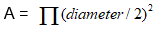=> l = R x A / ρ

=> l = (10 x 3.14 x 25) / (4 x 1.6)

On solving we get, l = 122.72 m.

To find the resistance when the diameter is doubled:

We use, R = ρ l/A

R = 1.6 × 10–8x 122.72 / 3.14 x (0.001/2)2

R = 250.2 x 10-2 Ω = 2.5 Ω

7. The values of current I flowing in a given resistor for the corresponding values of potential difference V across the resistor are given below –

I (amperes) 0.5 1.0 2.0 3.0 4.0

V (volts) 1.6 3.4 6.7 10.2 13.2

Plot a graph between V and I and calculate the resistance of that resistor.

Answer:

We plot the current on Y axis and the voltage on X axis. This graph is called as IV characteristic graph. The slope of this graph gives the value of resistance.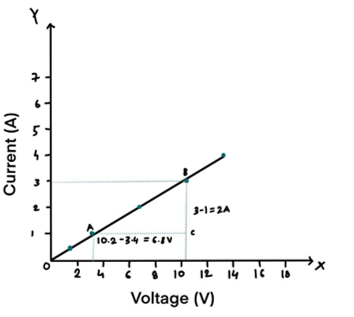Slope = 1/R = BC/AC = 2/6.8

To calculate R,

R = 6.8/2 = 3.4 Ω

The resistance of the resistor is 3.4 Ω.

8. When a 12 V battery is connected across an unknown resistor, there is a current of 2.5 mA in the circuit. Find the value of the resistance of the resistor.

Answer:

Value of the resistance is calculated by Ohm’s law.

R = V / I

R = 12 / 2.5 x 10-8 = 4.8 x 103 Ω

R = 4.8 kΩ

Hence, the value of the resistance of the resistor.

9. A battery of 9 V is connected in series with resistors of 0.2 Ω, 0.3 Ω, 0.4 Ω , 0.5 Ω and 12 Ω, respectively. How much current would flow through the 12 Ω resistor?

Answer:

When resistors are connected in series, the current flowing through them remains the same as there is no distribution of current.

The total resistance when the given resistances are connected in series is:

R = 0.2Ω + 0.3Ω + 0.4Ω + 0.5Ω + 12Ω = 13.4Ω

Now, we can use Ohm’s law to calculate the current flowing through the resistors:

I = V / R

I = 9 / 13.4

I = 0.671 A

Hence, we can say that a current of 0.671A flows through the 12Ω resistor.

10. How many 176 Ω resistors (in parallel) are required to carry 5 A on a 220 V line?

Answer:

Let the number of required resistor be ‘n’.

We know the total resistance when resistors are connected in parallel is:On putting 176Ω in the above equation and solving, we get:

=> RTotal = 176 / n = R

To calculate the ‘n’, we will use Ohm’s law:

R = V / I

176 / n = 220 / 5

n = 176 x 5 / 220

n = 4

Hence, we require four 176Ω resistors in parallel to carry 5A on a 220 V line.

11. Show how you would connect three resistors, each of resistance 6 Ω, so that the combination has a resistance of (i) 9 Ω, (ii) 4 Ω.

Answer:

(i)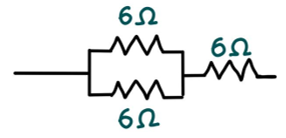The two parallel resistors give : 1/R = 1/6 + 1/6

R = 3 Ω

Combining with series we get: 3 Ω + 6 Ω = 9 Ω.

(ii)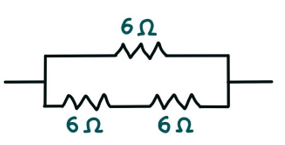Resistors in series give: 6 Ω + 6 Ω = 12 Ω

Adding another 6 Ω in parallel we get: 1/R = 1/6 + 1/12

R = 12 / 3 = 4 Ω

12. Several electric bulbs designed to be used on a 220 V electric supply line, are rated 10 W. How many lamps can be connected in parallel with each other across the two wires of 220 V line if the maximum allowable current is 5 A?

Answer:

Given: Power (P) = 10 W.

To find the resistance of one bulb we can use the formula:

P = V2 / R

=> R = V2 / P

=> R = (220)2 / 10

=> R = 4840 Ω

We can find the total resistance to be provided by ‘n’ number of bulbs by applying Ohm’s law:

Rtotal = V / I

Rtotal = 220 / 5

Rtotal = 44 Ω

Finally to find the number of bulbs with resistance 4840Ω required in parallel:1/Rtotal = n x 1/4840

n = 4840 / 44 = 110

Hence, we require 110 bulbs of 4840Ω resistance connected in parallel.

13. A hot plate of an electric oven connected to a 220 V line has two resistance coils A and B, each of 24 Ω resistance, which may be used separately, in series, or in parallel. What are the currents in the three cases?

Answer:

When the coils are used separately:

Since both have same resistance, using Ohm’s law we get:

I = V / R

I = 220 / 24

I = 9.166 A

When the coils are connected in series:

In series, total resistance = 24Ω + 24Ω = 48 Ω

Using Ohm’s law to find the current:

I = V / R

I = 220 / 48

I = 4.58 A

When the coils are connected in series:

When connected in parallel = 1/24 + 1/24

R = 576/48 = 12 Ω

Using Ohm’s law to find the current:

I = V / R

I = 220 / 12

I = 18.33 A

14. Compare the power used in the 2 Ω resistor in each of the following circuits: (i) a 6 V battery in series with 1 Ω and 2 Ω resistors, and (ii) a 4 V battery in parallel with 12 Ω and 2 Ω resistors.

Answer:

We define power (P) = I2R = V2 / R

(i) Since the given resistors are connected in series, the total resistance will be: 1Ω + 2Ω = 3Ω.

We can use Ohm’s law to find the current flowing through the resistors:

I = V / R

I = 6 / 3

I = 2 A

Now, using the obtained data to get the power used in 2Ω resistor:

P = (2)2x2

P = 8 W

(ii) Since the given resistors are connected in parallel, the potential difference across them remains the same:

P = V2 / R

P = 42 / 2

P = 8 W.

15.Two lamps, one rated 100 W at 220 V, and the other 60 W at 220 V, are connected in parallel to electric mains supply. What current is drawn from the line if the supply voltage is 220 V?

Answer:

Since the two lamps are connected in parallel, the voltage across them will remain equal.

To find the current drawn by the first lamp, we use the equation: P = V x I

=> I = V / P

=> I = 100 / 220 A

Similarly, for the second lamp:

I = 60 / 220 A

Hence, the total current drawn

I total = (100 / 220) + (60 / 220)

I total = 160 / 220 A.

I total = 0.727 A

Hence, 0.727 A of current is drawn from the line if the supply voltage is 220 V.

16. Which uses more energy, a 250 W TV set in 1 hr, or a 1200 W toaster in 10 minutes?

Answer:

We know; H = P x t

Where P = power and t = time in seconds.

Energy consumed by a 250 W TV set in 1 hr (3600 s) :

H = 250 W x 3600 s = 9 x 105 J

Energy consumed by a 1200 W toaster in 10 minutes (600s) :

H = 1200 W x 600 s = 7.2 x 105 J

We see that watching one hour of TV consumes more energy than using a toaster for 10 minutes.

17. An electric heater of resistance 8 Ω draws 15 A from the service mains 2 hours. Calculate the rate at which heat is developed in the heater.

Answer:

We know power is energy consumed per second.

Power P is given by : P = I2R

Given: R = 8 Ω and I = 15 A

P = (15)2 x 8

P = 1800 J/s

Hence, the rate at which heat is developed in the heater is 1800 J/s.

18. Explain the following.

(a) Why is the tungsten used almost exclusively for filament of electric lamps?

(b) Why are the conductors of electric heating devices, such as bread-toasters and electric irons, made of an alloy rather than a pure metal?

(c) Why is the series arrangement not used for domestic circuits?

(d) How does the resistance of a wire vary with its area of cross-section? (e) Why are copper and aluminium wires usually employed for electricity transmission?

Answer:

(a) Electric lamps operate at high temperatures. Since tungsten has a very high resistance and melting point, it does not melt at the temperatures on which the the lamps operate. Hence, tungsten used almost exclusively for filament of electric lamps.

(b) Since the resistance given by an alloy is much higher than that of pure metals, they are able to dissipate more heat. They also have higher melting points as compared to metal. Because of these two properties, the conductors of electric heating devices, such as bread-toasters and electric irons, made of an alloy rather than a pure metal.

(c) In series circuit, the voltage is divided across the resistors. As a result, each component in the circuit receives a small voltage because of which the amount of current decreases and the device generates a lot of heat and does not work properly or can be damaged. This is the reason why series circuits are not used in domestic circuits.

(d) Resistance is inversely proportional to the area of cross section.

R = ρ l/A

where,

ρ is the resistivity of the material of the wire,

l is the length of the wire

A is the area of the cross-section of the wire.

As the area of the cross section increases, the resistance decreases and vice-verse.

(e ) Copper and aluminium are good conductors of electricity and have low resistivity. These properties reduce the loss of energy in the form of heat.  Hence, they are usually employed for electricity transmission.

### Topics Covered in Electricity Class 10 Science

• Electric Current and Circuit
• Electric Potential and Potential Difference
• Circuit Diagram
• OHM’S LAW
• Resistance of conductors
• System Of Resistors
• Heating Effect
• Electric Power